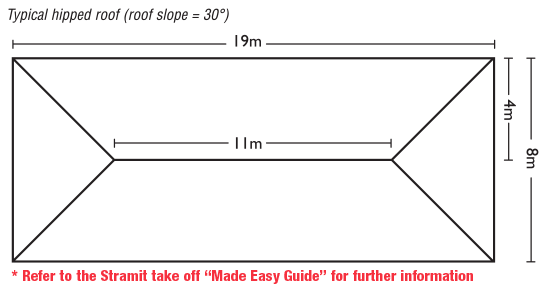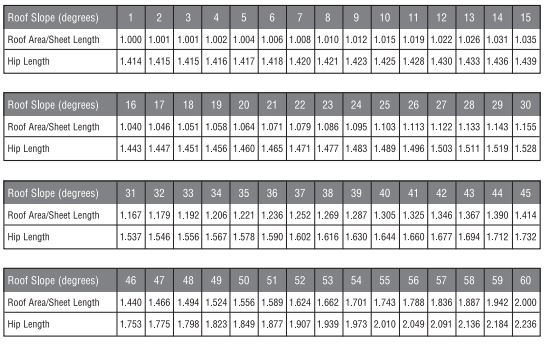PRODUCTSVisit
PROJECT GALLERYVisit
NEWSVisit
RESOURCESVisit

# Sometimes you need to do a quick calculation to see if the numbers stack up. We get it.

Our table of roof slope factors will help you do the maths when it comes to roof areas, sheet length and hip length. Remember, these methods are for quick estimating purposes only. Accurate measurements must be taken from the actual roof frame before ordering roofing and rainwater products.

As an example, take a typical hipped roof where all four roof sections have the same slope (30 degrees) and all hips are of equal length.### Roof area

First determine the area of the roof plan by multiplying the length of the roof by the width. Before making the calculation, add gutter overhangs of 50mm. To find the area of your hipped roof, simply multiply your plan area by the Roof Slope Factor for the roof area. The same method is used for gable roofs. If we apply our example, the equation is as follows:

19.1 x 8.1 = 154.7 x 1.155 = 178.7

Roughly 179m2 of sheeting will be needed to cover the roof. On complex roof shapes, extra may be needed to allow for wastage.

### Sheet length

On the roof plan, measure the distance from the line of the ridge to the outside of the fascia, which in the example we've provided is half the span or 4000mm. Multiply this measurement by the Sheet Length Roof Slope Factor to find the length of the sheet required to span from the ridge to the fascia. Note that all roof sheeting must extend at least 50mm beyond the fascia line to provide gutter overhang.

4000 +50 x 1.155 = 4678mm

### Hip capping

To find the length of ridge capping required to cover one hip, measure the distance from the ridge line to the fascia on the roof plan (here 4000mm) and the gutter overhang and multiply by the Hip Length Roof Slope Factor for 30 degrees. Multiply by 4.

4000 + 50 x 1.528 x 4 = 24.8m

### Ridge capping

For hipped roofs where all sides have the same slope, the length of the top ridge is equal to the length of the roof plan minus the width, regardless of slope. Add 5% to all cappings to allow for laps.

19 - 8 = 11 + 5% = 11.55m

### Roof slope factors